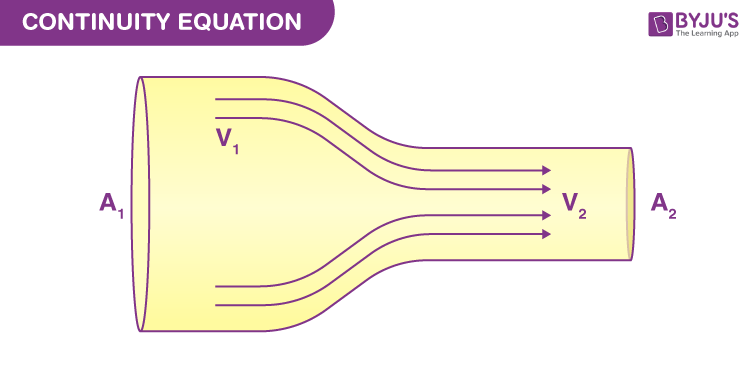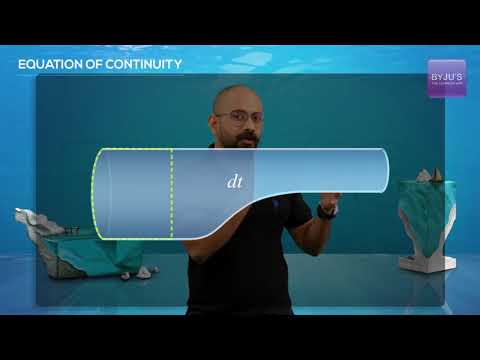# Continuity Equation

The transfer of various quantities, such as fluid or gas, is described by the continuity equation. The formula describes how a fluid conserves mass while moving. How a fluid conserves mass while moving is described by the equation. The continuity equations can be used to demonstrate the conservation of a wide range of physical phenomena, including energy, mass, momentum, natural numbers, and electric charge. The continuity equation offers useful knowledge about the flow of fluids and their behaviour as they go through a pipe or hose. The Continuity Equation is used on a variety of objects, including tubes, pipes, rivers, and ducts that carry gases or liquids. The continuity equation can be written in differential form, which is applied at a point, or in integral form, which is applied in a finite region.

### Deriving the Equation of Continuity$$\begin{array}{l}m = \rho _{i 1} \ v _{i 1} \ A _{i 1} + \rho _{i 2} \ v _{i 2} \ A _{i 2} + ….. + \rho _{i n} \ v _{i n} \ A _{i m}\end{array}$$

$$\begin{array}{l}m = \rho _{o 1} \ v _{o 1} \ A _{o 1} + \rho _{o 2} \ v _{o 2} \ A _{o 2} + ….. + \rho _{o n} \ v _{o n} \ A _{o m}……….. (1)\end{array}$$

Where,

m = Mass flow rate

ρ = Density

v = Speed

A = Area

With uniform density equation (1), it can be modified to:

$$\begin{array}{l}q = v _{i 1} \ A _{i1} + v _{i2} \ A _{i2} + …. + v _{i n} \ A _{i m}\end{array}$$

$$\begin{array}{l}q = v _{o 1} \ A _{o1} + v _{o2} \ A _{o2} + …. + v _{o n} \ A _{o m}………..(2)\end{array}$$

Where,

q = Flow rate

## Fluid Dynamics

According to the continuity equation in fluid dynamics , the rate at which mass exits a system is equal to the rate at which mass enters a system in any steady state process.
The differential form of the continuity equation is:

$$\begin{array}{l}\frac{\partial \rho}{\partial t} + \bigtriangledown \cdot \left (\rho u \right) = 0\end{array}$$

Where,

t = Time

ρ = Fluid density

u = flow velocity vector field.

Watch the video to learn more about the practical application of the equation of continuity, its derivation, fluid velocity and different types of flow: steady flow and turbulent flow.## Frequently Asked Questions – FAQs

Q1

### What is the principle of continuity?

The continuity equation describes the transport of some quantities like fluid or gas. For example, the equation explains how a fluid conserves mass in its motion. Many physical phenomena like energy, mass, momentum, natural quantities, and electric charge are conserved using the continuity equations.
Q2

### Where is the Equation of Continuity used?

The continuity equation provides very useful information about the flow of fluids and their behaviour during their flow in a pipe or hose. Continuity Equation is applied on tubes, pipes, rivers, ducts with flowing fluids or gases and many more.
Q3

### What is the importance of the continuity equation?

The continuity equation proves the law of conservation of mass in fluid dynamics.
Q4

### What does the continuity equation in fluid dynamics describe?

The continuity equation in fluid dynamics describes that in any steady-state process, the rate at which mass leaves the system is equal to the rate at which mass enters a system.
Q5

### When can the Continuity equation be used?

The continuity equation applies to all fluids, compressible and incompressible flow, Newtonian and non-Newtonian fluids.

Register to “BYJU’S – The Learning App” for loads of interactive, engaging Physics-related videos and unlimited academic assist.

Test Your Knowledge On Continuity Equation!